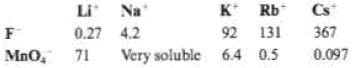# Explain the trends in solubility (grams per 100 mL of water) of the alkali-metal fluorides and permanganates.### General Chemistry - Standalone boo...

11th Edition
Steven D. Gammon + 7 others
Publisher: Cengage Learning
ISBN: 9781305580343### General Chemistry - Standalone boo...

11th Edition
Steven D. Gammon + 7 others
Publisher: Cengage Learning
ISBN: 9781305580343

#### Solutions

Chapter
Section
Chapter 12, Problem 12.46QP
Textbook Problem

## Explain the trends in solubility (grams per 100 mL of water) of the alkali-metal fluorides and permanganates.Expert Solution
Interpretation Introduction

Interpretation:

The trend observed in solubility of fluoride and permanganate salts of alkali metals has to be discussed.

Concept Introduction:

The energy released when gaseous state ions of unlike charges that are infinitely farther apart combine to form a stable ionic solid is called Lattice energy.  Conversely, the energy required to break the electrostatic force of attraction between the ions of unlike charges in the ionic solid and revert them to gaseous state is also termed as Lattice energy of an ionic solid.

Hydration energy refers to the quantity of energy released when one mole of ion gets hydrated at constant pressure.  Hydration energy of an ion depends upon its ionic size.

### Explanation of Solution

Fluoride anions are smaller in size.  Greater the lattice energy of an compound, higher will be the energy of hydration and more soluble the compound.  As the cationic radii increases, lattice energy of the fluoride compounds decreases from Lithium to Cesium fluoride because the internuclear distance between the smaller fluoride anion and larger metal ion increases the same way.  Decrease in lattice energy leads to decrease in hydration energy that the fluoride compounds become less soluble from Lithium to Cesium...

### Want to see the full answer?

Check out a sample textbook solution.See solution

### Want to see this answer and more?

Bartleby provides explanations to thousands of textbook problems written by our experts, many with advanced degrees!

See solution